Mathematics

# Bar Plots and Line Graph Types

492 views

 1 Introduction to Graphical Data 2 Types of Graphical Representations 3 Bar Graphs & its types 4 Line Graphs & its types 5 Applications in Real Life 6 Summary 7 FAQs

02 November 2020

## Introduction to Graphical Data

When you collect and record data, you'll represent it during a diagram. To point out the results, you'll use a bar graph, pie chart, line graph, pictogram, frequency diagram, or scatter diagram. Graphs are nothing but the chart that helps us to convey the knowledge and in fact to form a sense of the info. A graph of various kinds is often applied in some ways.

## Types of Graphical Representations

### Line graph

A line graph could even be a singular graph, which is usually utilized in statistics. It represents the change during a quantity with reference to another quantity This variation is typically plotted during a two-dimensional XY plane. If the relation, including any two measures, is often expressed utilizing a line during a graph, then such graphs are called linear graphs. Thus, the road graph is additionally called a linear graph. A line graph could even be a graph that utilizes points and features to represent change over time. It is a chart that shows a line joining several points or a line that shows the relation between the points. Linear graphs compare these

variables during a vertical axis and a horizontal axis.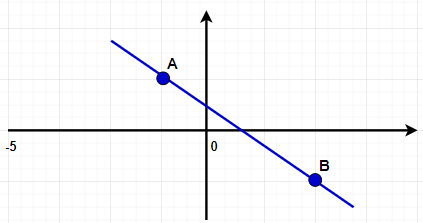### Bar graph

A bar graph is employed to match two or more values with a little set of results.

In a bar graph, the peak of the bar shows the frequency of the result. A bar chart is one that presents categorical data having rectangular bars with heights or lengths proportional to the values which they're representing. We will plot the bars horizontally or vertically. We also ask for a vertical bar graph to also as a column chart.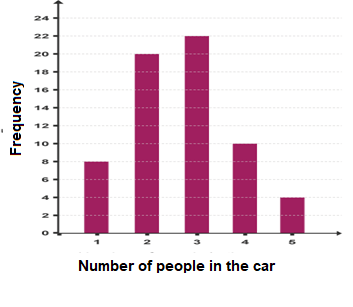### Pictograms

Pictograms use pictures to represent data. To form a sense, a pictogram should have a key. In a pictogram, it's important to form sure that every picture is the same size, equally spaced out, and lined up one beneath the opposite.

This pictogram shows the number of pizzas eaten by four friends within the past month: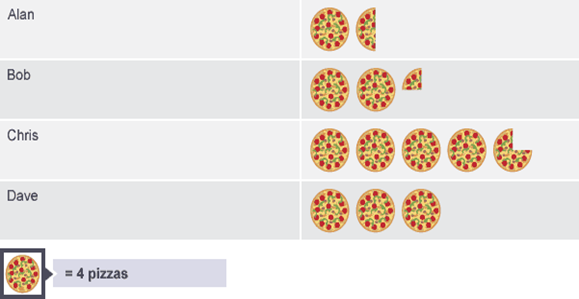### Pie charts

Pie charts use different-sized sectors of a circle to represent data. A chart may be a circle that is split into a variety of parts.

In a chart, it's important to know that the angle of every sector represents the fraction, out of, assigned thereto data value. Pie charts should be labeled, either directly on the chart or by means of a color-coded key.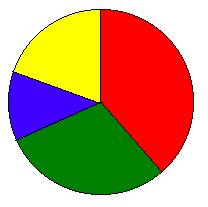This chart shows the results of a survey to seek out out how students visit the school: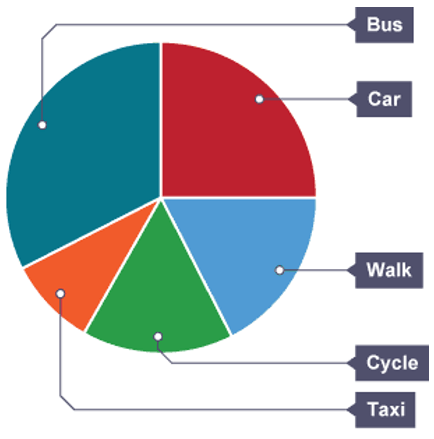### Frequency diagrams and polygons

Frequency polygons are particularly useful for comparing different sets of knowledge on an equivalent diagram.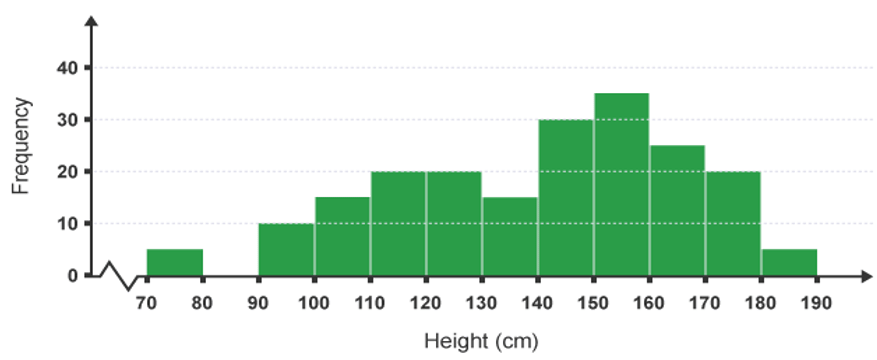### Scatter diagrams

These are used to compare two sets of knowledge. One set of knowledge is placed on the x-axis (the horizontal axis) and, therefore the other on the y-axis (the vertical axis). If one set of knowledge depends upon the opposite, this is often placed on the y-axis (and is understood because of the 'dependent variable'). For instance, if you were plotting a child's height at various times, the peak would depend on the time. The peak is that variable and goes on the y-axis, whereas time doesn't depend upon anything, then is that the experimental variable and goes on the x-axis.

Usually, we are looking to ascertain if there's a relationship between the 2 sets of knowledge. We draw a line of best fit. This could have roughly an equivalent number of points above and below it.

The less scatter there's about the best-fit line, the stronger the connection is between the 2 quantities. If the points are on the brink of the best-fit line, we are saying that there's a robust correlation. If the points are loosely scattered, there's a weak correlation. We are saying there's zero correlation if there's no linear relationship between the variables- in other words if we will not draw a meaningful best fit line.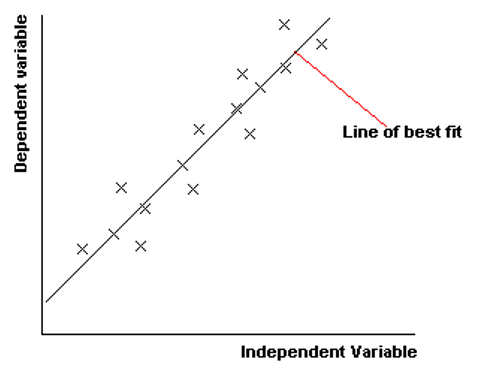Also, if the simplest fit line slopes upwards, love it does below, then the items we are comparing go up together. We are saying that there's a direct correlation. If the road slopes down, the 'dependent variable' decreases because of the 'independent variable' increases. We are saying there's an indirect correlation.

The English and Maths results of ten classmates are shown within the table below: To see whether there's a correlation between the Maths marks and, therefore the English marks, you'll plot a scatter diagram.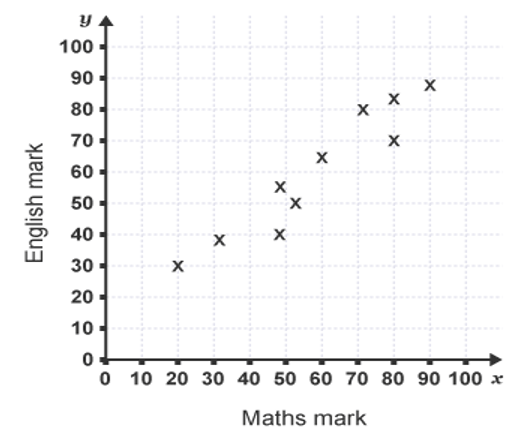Notice that each one of the points representing Maths marks and English marks lie approximately along a line. This shows that there's a correlation between these two variables. The diagrams below show the correlations that you simply can deduce from different patterns of scatter plot.

- Positive correlation

The points lie on the brink of a line, which features a positive gradient.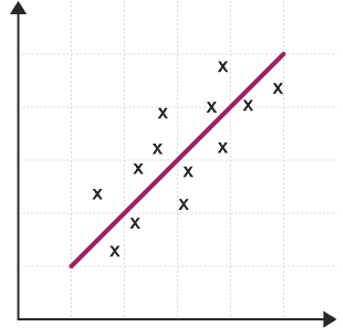This shows that together variable increases the opposite increases.

- Negative correlation

The points lie on the brink of a line, which features a negative gradient.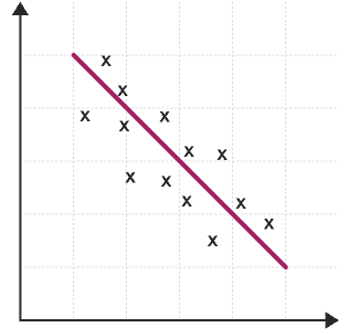This shows that together variables increase, the opposite decreases.

#### - No correlation

The points don't lie on the brink of any line.This shows that there's no connection between the 2 variables.

## Bar Graphs & its types

### Properties of Bar Graphs

Each bar or column during a bar chart is of equal width. All bars have a standard base. The height of the bar corresponds to the worth of the info. The distance between each bar is the same.

### Construction of a bar chart

Draw two perpendicular lines intersecting one another at some point O. The vertical line is that of the y-axis, the horizontal is that of the x-axis. With the bar chart, we will also compare the marks of scholars in each subject aside from the marks of 1 student in every subject. Also, we will draw the bar chart for each student altogether. Choose an appropriate scale to work out the peak of every bar.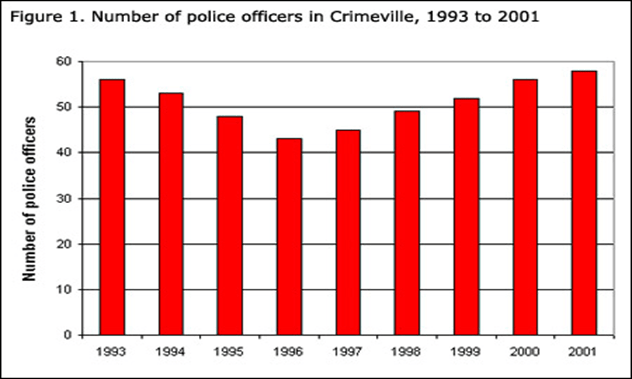At the horizontal line, draw the bars at equal distance with corresponding heights.

The space between the bars should be equal.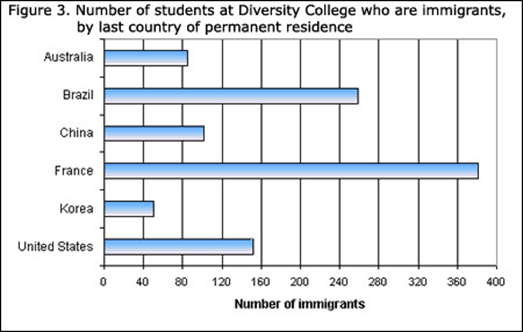A bar chart is often either vertical or horizontal depending upon the selection of the axis because of the base. Let us take an example for a bar chart showing the comparison of marks of a student altogether subjects out of 100 marks.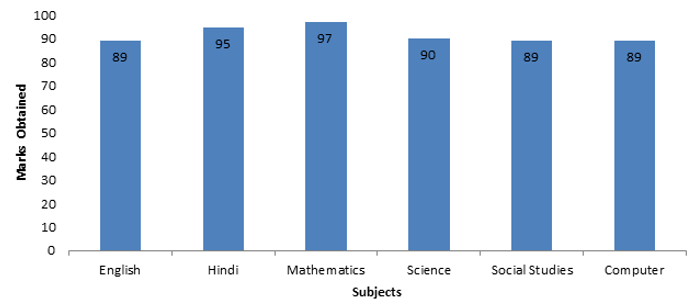## Line Graphs & its types

### Types of Line Graphs

The following are the kinds of line graphs:

1. Simple Line Graph: Just one line is plotted on the graph.

2. Multiple Line Graph: Multiple one lines are plotted on an equivalent set of axes. A multiple line graph can effectively compare similar items over an equivalent period of a while.

3. Compound Line Graph: If information is often subdivided into two or more sorts of data. This sort of line graph is understood as a compound line graph. Lines are drawn to mean a neighborhood of a whole. The absolute best line shows the entire and the line below shows an area of the entire. The space between every two lines shows the dimensions of every part.

4. Vertical Line Graph: Vertical line graphs are graphs during which a vertical line extends from each datum right right right down to the horizontal axis. A line parallel to the y-axis is understood as a vertical line.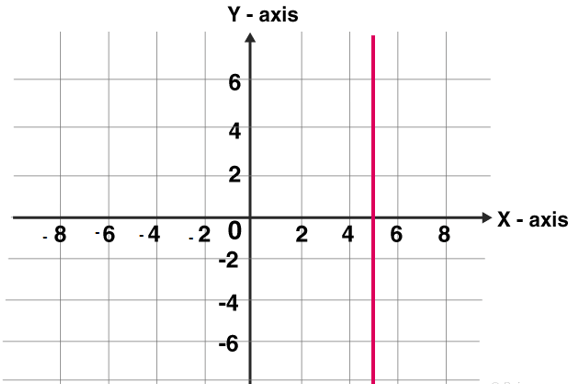5. Horizontal Line Graph: Horizontal line graphs are graphs during which a horizontal line extends from each datum parallel to the earth. A line parallel to the x-axis is understood as a vertical line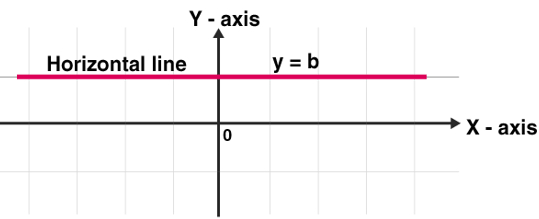6. Straight Line Graph: A line graph could even be a graph formed by segments of straight lines that join the plotted points that represent given data. The road graph is employed to unravel changing conditions, often over a selected interval. A general linear function has the shape y = mx + c, where m and c are constants.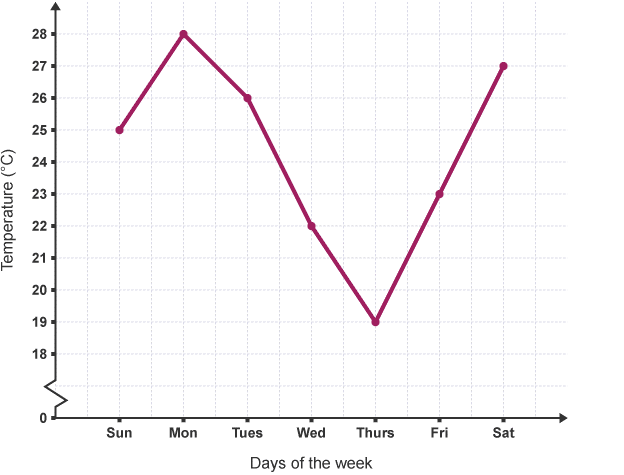We require only two points to graph a line. The subsequent procedure is followed in drawing linear graphs:

By substituting two dissimilar values for x within the equation y = mx + c, we get two values for y. Thus, we get two points (x1, y1) and (x2, y2) on the road.

Plot the horizontal line and vertical line and select the acceptable scale for both the axis.

Suppose the given table values are large to choose the size for that specific value. It depends on the given value.

Plot the 2 points within the plane of the paper. Join the 2 points employing a line segment and reach two directions. The closed figure was obtained; it's the required linear graph.

### Different Parts of Line Graph

1. Scale: the size is that the numbers that specify the units utilized on the linear graph.
2. Labels: Both the side and thus the lowest of the linear graphs have a label that indicates what quiet data is represented within the graph. X-axis describes the info points on the road and thus, the y-axis shows the numeric value for every point on the road.
3. Bars: They measure the info number.
4. Data values: they're the particular numbers for every point.

### How to Make a Line Graph?

If we've created data tables, then we draw linear graphs using the info tables. These graphs are plotted as a series of points, which are later joined with straight lines to supply an easy due to review data collected over time. It offers an impressive visual format of the result data collected over time.

To draw a linear/line graph follow the below steps:

1. Use the info from the data-table to settle on an appropriate scale.
2. Draw and label the size on the vertical (y-axis) and horizontal (x-axis) axes.
3. Name each item and place the points on the graph.
4. Join the points with line segments.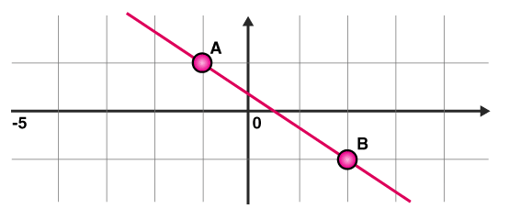### Double Line Graph

A double line graph could even be a line graph with two lines. A graph that compares two different subjects over a period of a while. A double line graph tells how things change over a period of a while. The double line graph tells two line graphs within one chart. Double line graphs are wont to compare trends and patterns between two subjects.

#### Steps to form a Double Line Graph:

1. Use the info from the table to settle on an appropriate scale.
2. Draw and label the size on the vertical and horizontal axis.
3. Name each item and locate the points on the graph for both the lines.
4. Attach the points with line segments separately of both the lines.
5. Make two line graphs within one chart. The points on the double line graph show the quality monthly rainfall within the two cities (city 1, city 2). The separate lines that are made by connecting the points for every city.

The important use of line graphs is to trace the changes over the short and long period of a while. It also wants to compare the changes over an equivalent period of a while for various groups. It’s often better to use the road than the bar chart, whenever the tiny changes exist. It always represents the quantity of some time of the info.

## Applications in Real Life

The scatter plot is a superb quick analytic tool. Watching a mass of knowledge, I’m wondering if two variables are related or not, and, if they're, what the connection is. I quickly dump the info into a scatter plot as a primary step. It’ll tell me if there's any correlation worth investigating. If the scatter is a filled-in circle of dots, then there's no correlation. If I see an upward or downward line, there's a linear correlation. More complex correlations produce other shapes.

Here may be a real-world example. Suppose a faculty runs from 1st to 12th grade. They think they're an above-average school. They need the many students on a uniform test just like the SAT or the CAT for each graduating senior, and that they also know the number of years that senior was in their school. (Some students started in class. Others came to the varsity in later years.) A scatter plot would show if there's a correlation between the number of years during this school and, therefore the SAT or CAT score.

Here's a true case where a scatter plot would have provided fast data analysis. A bakery is barely breaking even. they have to extend revenue to remain in business. They create a knowledge set correlating day of the week and hour of the day with total dollar sales therein hour. So, if they open weekdays 7 am to 6 pm, they now earn a lot of money each hour of the day, and every day of the week.

They do a scatter plot on this. They discover an odd dip - there's much less revenue the primary 3 hours every Tuesday morning. They check to ascertain why. They discover that what makes Tuesday different is that the toilet is cleaned before the shop opens on Tuesday morning, and therefore the cleansers don't leave a pleasing smell. they modify the routine and clean the toilet within the evening before the shop closes, and sales go up and therefore the company stays in business.

## Summary

A picture tells a thousand words. 90% of the knowledge sent to the brain is visual and over 90% of all human communication is visual. Processing text requires our brains to figure much harder than when processing images.

There is considerable truth within the saying ‘a picture tells a thousand words’. it's going to not be literally a thousand, but it's often much easier to use an image than to explain numerical information in words.

The data itself could also be vitally important, but without a visible presentation of that data, its impact (and therefore your message) could also be lost.

There are many of us within the world who don't find it easy to know numbers.

There also are many of us who will simply cut if you show them figures during a table. But if you present data during a graph or chart, you create a picturing of the info. It makes the numbers much easier to know. Trends and proportions become more obvious. Even for the highly numerate, the immediate point is merely that there are a lot more sales within the half-moon. you'd need to do some adding up and dividing to figure out the relationships between the four numbers. It also requires far more concentration to read and absorb the knowledge during this format. It is immediately and shiningly obvious, even for those that struggle with numbers, that quite half all sales were within the half-moon, which over 75% were within the first two quarters.

What’s more, nobody goes to be straining from the rear of the space to read your figures. you actually can see tons more from an image.

But, and this is often important, confirm that the graph may be a good one.

## What is data?

Data may be a collection of facts, like numbers, words, measurements, observations or simply descriptions of things.

## How do you differentiate between data and information?

Data is raw, unorganized facts that require to be processed. Data is often something simple and seemingly random and useless until it's organized. When data is processed, organized, structured, or presented during a given context so as to make it useful, it's called information.

Example: Each student's test score is one piece of knowledge. The typical score of a category or of the whole school is information that will be derived from the given data.

## What are the ways to represent data?

There are a variety of ways of representing data diagrammatically. The solution is that you simply should use figures and numbers whenever they provide the simplest evidence to copy your argument or to inform your story.

## What are the different types of graphs?

• Line graph
• Bar graph
• Pictograms
• Pie charts
• Frequency diagrams and polygons
• Scatter diagrams

## What are the different types of bar graphs?

• Vertical bar graphs
• Horizontal bar graphs
• Straight bar graph

## What are the different types of line graphs?

• The following are the kinds of line graphs:
• Simple Line Graph: just one line is plotted on the graph.
• Multiple Line Graph: Only one line is plotted on an equivalent set of axes. A multiple line graph can effectively compare similar items over an equivalent period of your time.
• Compound Line Graph: If information is often subdivided into two or more sorts of data. This sort of line graph is called a compound line graph.
• Lines are drawn to point out the part of a complete. The highest line shows the entire, and the line below shows a part of the whole. The space between every two lines shows the dimensions of every part.

Award-winning math curriculum, FREE for a year